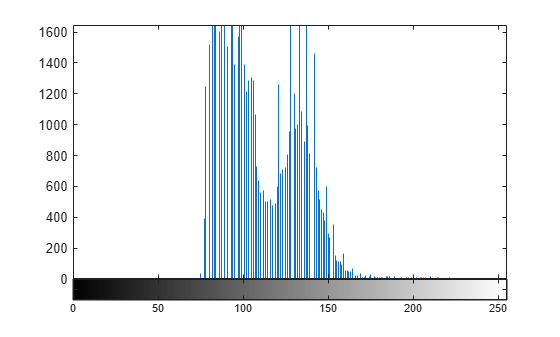imhist

Histogram of image data

Description

[counts,binLocations] = imhist(I) calculates the histogram for the grayscale image I. The imhist function returns the histogram counts in counts and the bin locations in binLocations. The number of bins in the histogram is determined by the image type.

[counts,binLocations] = imhist(I,n) specifies the number of bins, n, used to calculate the histogram.

[counts,binLocations] = imhist(X,cmap) calculates the histogram for the indexed image X with colormap cmap. The histogram has one bin for each entry in the colormap.

example

imhist(___) displays a plot of the histogram. If the input image is an indexed image, then the histogram shows the distribution of pixel values above a color bar of the colormap cmap.

Examples

collapse all

Read a grayscale image into the workspace.

Display a histogram of the image. Since I is grayscale, by default the histogram will have 256 bins.

imhist(I)Display the histogram of the data. Since the image is grayscale, imhist uses 256 bins by default.

imhist(mristack)Input Arguments

collapse all

Grayscale image, specified as a numeric array of any dimension. If the image has data type single or double, then values must be in the range [0, 1]. If I has values outside the range [0, 1], then you can use the rescale function to rescale values to the expected range.

Data Types: single | double | int8 | int16 | int32 | uint8 | uint16 | uint32 | logical

Number of bins, specified as a positive integer. If I is a grayscale image, then imhist uses a default value of 256 bins. If I is a binary image, then imhist uses two bins.

Example: 50

Data Types: single | double | int8 | int16 | int32 | int64 | uint8 | uint16 | uint32 | uint64

Indexed image, specified as a numeric array of any dimension.

Data Types: single | double | uint8 | uint16 | logical

Colormap associated with indexed image X, specified as a c-by-3 numeric matrix with values in the range [0, 1]. Each row is a three-element RGB triplet that specifies the red, green, and blue components of a single color of the colormap.The colormap must be at least as long as the largest index in X.

Data Types: double

Output Arguments

collapse all

Histogram counts, returned as a numeric array. If the histogram is computed for an indexed image, X, then the length of counts is the same as the length of the colormap, cmap.

Bin locations, returned as a numeric array.

Tips

• For grayscale images, the n bins of the histogram are each half-open intervals of width A/(n−1). In particular, the pth bin is the half-open interval

$\frac{A\left(p-1.5\right)}{\left(n-1\right)}-B\le x<\frac{A\left(p-0.5\right)}{\left(n-1\right)}-B,$

where x is the intensity value. The scale factor A and offset B depend on the type of the image class as follows:

Data TypeAB
double10
single10
int8255128
int1665,53532,768
int324,294,967,2952,147,483,648
uint82550
uint1665,5350
uint324,294,967,2950
logical10

• To display the histogram from counts and binLocations, use the command stem(binLocations,counts).